occasional meanderings in physics' brave new world

## Friday, February 29, 2008

### Damned Numbers

Carl and kneemo, amongst others, like to think about that damned number, otherwise known as the phase angle determining the charged lepton mass matrix, which is

$\phi = 0.22222204717$

to within experimental precision: notably close to $\frac{2}{9}$. The $3 \times 3$ MUB problem says nothing about this phase. Since phases usually involve factors of $\pi$, one wonders if there are any well known numbers that, when multiplied by $\pi$, also give numbers very close to $\frac{2}{9}$. For example, consider the first zero of the Riemann zeta function, namely $\gamma_{1} = 14.134725142$. Observe that

$\frac{\pi}{\gamma_{1}} = 0.222260611(5)$

which differs from $\frac{2}{9}$ by a factor of 1.000172751(75). So we didn't really need to look far to find a number satisfying this curiosity. Are there better ones?

### M Theory Lesson 163

I was delighted to come across a remarkable paper by Bolker, Guillemin and Holm, entitled How is a graph like a manifold? The paper begins with some remarks on Stanley's proof of McMullen's conjecture and then moves onto geometric definitions and problems involving the Betti numbers of graphs.

For example, the graph of the symmetric group $S_{n}$, that is the permutohedron, corresponds to the flag manifold of subspaces of $\mathbb{CP}^{n}$. In M Theory we are particularly interested in $S_{3}$, a hexagon including the diagonals, which has three geodesics, one of which is shown in the diagram.## Thursday, February 28, 2008

### M Theory Lesson 162

As a zonohedron, the 3 dimensional permutohedron is generated by the 12 edges of the octahedron, which become the edges shared by two hexagons.In this scheme, the required list of 6 generators (edges come in pairs) is

{1,1,0} {1,-1,0} {1,0,1} {1,0,-1} {0,1,1} {0,1,-1}

which corresponds to two $3 \times 3$ circulant sets. Now to obtain the cube from the permutohedron, observe that the 8 hexagons are vertices, the 6 squares are faces, and the 12 special edges ($\frac{1}{3} \times 36$) are the edges. The incidence relations for a cube may be read off the permutohedron:

* each hexagon touches 3 squares
* each edge joins 2 squares
* each edge lies on 2 hexagons

and so on. Note also that the 24 vertices represent 3 times the number of vertices of a cube, because each of 6 vertices of a hexagon is shared by 2 hexagons.

### Against Symmetry II

Carl might not like the term background independence, but I have fond memories of independently lurking in the dark corners of a Sydney library in the early '90s, trying to figure out how quantum groups might allow us to throw out classical spaces in our construction of operators. I gave some seminars on applications of quantum groups to high temperature superconductivity, but these were no doubt naive and no one in the audience paid the least attention.

Not much has changed, although with the net I can now lurk from the comfort of home. Sometime in 1995, I decided that the early quantum group papers were trying to put spaces underneath the algebras, just like in old fashioned gauge theory. And even today, some people insist on pointing out that operads must act on something. Fortunately, at this level of abstraction, we can say that the spaces concerned are models or ideas imposed as experimental constraints, and they are not supposed to represent an actual aether, appearing universally in any question we might think to ask.

## Tuesday, February 26, 2008

### M Theory Lesson 161

The permutohedron in three dimensions is the octahedron with truncated vertices, which form six square faces between eight hexagons.Recall that the octahedron is dual to a cube, which in turn gives the permutohedron via the Loday maps. That is, the permutohedron (and the associahedron) acts as an intermediary between the two dual objects. Beneath duality lurks the shadow of triality!

## Monday, February 25, 2008

### M Theory Revision

Let us recall that one familiar instance of a hexagon represents the paths in a cube, labelled by monomials in $X$, $Y$ and $Z$.Edges of the hexagon must represent the morphing of one path into another, which is to say a face of the cube. Observe how a 1-circulant and a 2-circulant label three vertices each. We could teach this in kindergarten.

## Sunday, February 24, 2008

### Against Symmetry

Kostant's talk on Lisi's $E8$ physics appears to have renewed interest in the idea of One Big Group to explain everything. But symmetry, on its own, explains nothing at all. The 20th century idea that (standard) model building has sufficient explanatory power in itself is hopelessly inadequate for tackling the problems of quantum gravity. Lots of smart people tried this idea (Lie group based GUTs) and they failed. Did anyone notice? This idea FAILED!

Consider some basic examples of symmetry groups and their representations: say rotations of a sphere. One easy way to shift to a larger group is to increase the dimension of the sphere. But in doing so, observe that nothing in the underlying geometry of the space has been enriched. Whatever the dimension of $\mathbb{R}^{n}$, the symmetry rules for a sphere rely on the basic properties of the real numbers, analysis and the axioms of set theory and topology. In this scheme, what is the symmetry group of a point? You don't know? Shouldn't we actually understand this if we want our spaces to be associated with physical spacetime and matter's internal degrees of freedom?

I am sure Lisi appreciates that his paper is not a final explanation of how to unify the SM with gravity, but rather a new direction to probe effective descriptions of SM particle fields. So where does $E8$ really come from? Maybe $E8$ is pure moonshine...

## Saturday, February 23, 2008

### Housekeeping

Apparently the arxiv is now accepting Word documents. Clearly, they need to cater to the formatting preferences and abilities of all those permitted to post papers there. Meanwhile, Pioneer One writes about the ineffectiveness of peer review, and there is a wonderful mathematics carnival at JD2718, where AF is honoured to be included in the category Meta-topic.

## Friday, February 22, 2008

### M Theory Lesson 160

Monstrous moonshine tells us that the 1-ordinal indexing of the j invariant by powers of $q$

$j = q^{-1} +744 + 196884 q + 21493760 q^{2} + \cdots$

is roughly associated to the 1-ordinal indexing of an operad, since the coefficients are dimensions of the Monster modules $V_{i}$, which form an operad algebra. When interpreted as a lattice theta function, this indexing corresponds to the lengths of lattice vectors.

This correspondence between distance from the origin and dimension crops up in many unexpected quarters. For instance, in the method of geometric quantisation, the representations of $SU(2)$ are given by discretely spaced spheres in the dual of the Lie algebra for the group, basically $\mathbb{R}^{3}$. In fact, root lattices are just like this, living in the dual space to the Cartan algebra.

Well, it's probably time to mention E8 again: not only does the j invariant label a single node of an E8 diagram, but as Gannon points out, the dimension of E8 also appears in a single term of the expansion

$j^{\frac{1}{3}} = q^{\frac{-1}{3}} (1 + 248 q + 4124 q^{2} + 34752 q^{3} + \cdots )$

which is the generating function for the modular congruence group $\Gamma (3)$. It's neat that $4124 = 1031 \times 2^{2}$ has a simple prime factorisation, just like $248 = 31 \times 2^{3}$ (as Kostant mentions) and $34752 = 181 \times 3 \times 2^{6}$. I wonder why?

## Thursday, February 21, 2008

### M Theory Lesson 159

The PF thread discussing Lisi's work (and the E8 talk by Kostant) contains a link to Baez's page on some interesting remarks by McKay, alluded to by Gannon (see here). McKay says:
There is a remarkable connection between the three groups: Monster, 2.Baby Monster and 3.F24, and the graphs for E8, E7, and E6 respectively ... We may [alternatively] work with the folded Dynkin graphs of type E8, F4, G2 and collapse the centre. By moonshine, this means that each node of these extended graphs can be labelled by a modular function given by the moonshine correspondence, with the elliptic modular function j(z) corresponding to the identity of the Monster and so to the affine node of the E8 Dynkin graph. There is a similar interpretation for the other nodes. Adjacency is not understood but there should be a mechanism for obtaining the neighbours of a moonshine modular function occurring here.

## Tuesday, February 19, 2008

### Mutual Unbias VI

Here are the slides for a talk by the computer scientist Ross Duncan on MUBs at the workshop on Categories, Logic and Physics at Imperial College in January. No, I did not know about this before today's announcement about the next workshop.

## Monday, February 18, 2008

### Mutual Unbias VThe MUB literature review continues with a paper by Planat et al, containing this beautiful diagram of a Fano plane lying inside a cube, describing the geometry of operators for a system of two spin $\frac{1}{2}$ particles. This paper moves away from fields to look at projective geometry for Galois rings.

## Sunday, February 17, 2008

### Mutual Unbias IV

Thanks to Phil for the link to this recent seminar by A. Ericsson on MUBs and Hadamard matrices. The seminar looks at geometric aspects of the MUB problem, and its connection with more well known combinatorial problems. For example, the $3 \times 3$ circulant with entries $1,2$ and $3$ appears as a Latin square.

Ericsson's construction considers the $d+1$ bases as a polytope defined by the convex hull of points lying on certain simplices (with $d$ vertices defining a basis) in $(d-1)$ dimensional planes in the quantum state space (or rather, a density operator space) of dimension $d^2 - 1$, which is to say the space of $d \times d$ Hermitian matrices of trace 1. For example, when $d=2$, the polytope is the octahedron on a Bloch sphere (didn't that polytope come up just the other day?).

The problem of fitting the regular simplices into the polytope is shown to be equivalent to finding $d-1$ orthogonal Latin squares! This is the same as finding a finite affine plane of order $d$.

## Saturday, February 16, 2008

### Eleven

This paper by Kostant (a great mathematician who is currently busy giving seminars on Lisi's E8) mentions the $11 \times 11$ circulant matrix (associated to a $12 \times 12$ Hadamard matrix) with first row

$1, −1 ,−1, 1, −1, −1, −1, 1, 1, 1, −1$

The paper looks at $PSL(2,11)$ for the finite field $\mathbb{F}_{11}$, which is a little larger than the 5 or 7 that we are used to, but also interesting. One can make a planar geometry for this group, like the Fano plane, but with 11 lines.

## Thursday, February 14, 2008

### Ternary Geometry II

Recall  that the pullback $U \cap V$ of open sets in a manifold $M$ is essential to the definition of homology, which begins with the differential forms functor $\Omega^{\ast}$ acting on the arrows relating $M$ to the disjoint union of $U$ and $V$, in which the set $U \cap V$ is included. From the point of view of logic, it is a nice feature of manifolds that they are defined in terms of glued sets.

Let us view the Euler characteristic of a space as a homological entity (which it is). For a compact oriented manifold, under geometric Poincare duality the homology groups, and cohomology groups in the dual dimension, are isomorphic. Since the Euler characteristic is an alternating sum of dimensions of homology groups, it behaves simply under duality. Considering a compact hypersurface, in odd dimension $d$ we see that

$\chi = n_0 - n_1 + n_2 \cdots + n_d$

goes to $- \chi$ under duality. These two can only be equal if $\chi = 0$, which is the general result. Thus $\chi$ is not always a useful invariant. Its essence is already captured in dimension one, where $\chi = n_0 - n_1 = P - L$.

Observe that here we see only 2-logos (binary) logic, rather than ternary logic. Moreover, quantum invariants need not take values as scalars, but rather as q-numbers, perhaps represented by matrices. Now let us reinterpret the 2-logos $\chi$ as a combination of the Pauli MUB operators $1$ and $\sigma_{x}$ (the swap circulant, interpreted as -1).

A ternary analogue for $\chi$ is then naturally the $3 \times 3$ quantum Fourier transform, an example of which are the neutral and charged lepton mass matrices. Under triality, these matrices are invariant, at least up to equivalence.

 R. Bott and L. W. Tu, Differential Forms in Algebraic Topology, Springer (1982)

### M Theory Lesson 158

In lecture 25, Baez looks at the functor +1 on the groupoid of finite sets. Recall that addition is the disjoint union of sets, so this functor takes a finite set and adds a one point set to it. Now we can define groupoidified creation and annhilation operators.First take the span shown on the left. Applying the zero homology functor will turn the arrow +1 into the (groupoidified) creation operator. Similarly, starting with the functor +1 on the left yields the annihilation operator. The commutation relation between $a$ and $a^{\dagger}$ follows from considering the number of ways to take things out of a set and put them back in again. Think about it. Note that the composition of spans (the pink arrows) is given by a (weak) pullback, which conveniently exists for this category. Pullbacks allow products of arbitrary numbers of creation and annihilation operators.

Back down inside FinSet, recall that a pullback of two subspace arrows $U$ and $V$ is the intersection $U \cap V$. But in the groupoid FinSet0 the only arrows are bijections, and such limit diagrams do not exist. The finite set with $n$ elements has no arrow connecting it to the finite set with $n + 1$ elements. Thus the functor +1 acts simply as a categorified successor arrow for the ordinals.

Although not a satisfactory quantum gravitational definition for creation and annihilation, this approach goes some way towards giving a canonical diagrammatic representation for these operators. As Carl has pointed out, the Feynman diagrams for density matrix QFT should have an interpretation as products of creation and annihilation operators, a la Schwinger.

## Wednesday, February 13, 2008

### Mutual Unbias III

It turns out that Wootters, one of the MUB pioneers, gave a talk at a 2005 PI conference, at which Coecke also gave an interesting talk on categorical quantum mechanics. Highly recommended.

## Monday, February 11, 2008

### Ternary Geometry

In classical geometry, duality is a basic feature of the axioms. For 2 dimensional marked surfaces, swap points for face elements and lines for lines. Since the simplest concept of dimension is totally ordered, in an $n$ dimensional space one can always swap points for $n$-faces, lines for $(n-1)$-faces, and so on.

What would a ternary analogue of projective duality look like? Even without fixing upon axioms, it is clear that the ordered nature of dimension cannot so easily accommodate a ternary transformation between points and lines and surface elements. That is, we would like to consider a triality which takes points to lines, lines to faces, and faces to points. So we choose to generalise dimension to values in the higher ordinals, as necessary. First, however, let us consider the case of points, lines and faces with assigned dimensions of 0,1 and 2 respectively.

Are there any obvious collections of 0, 1 and 2 cells that allow for such ternary transformations?Consider the sphere with 2 hemispheres, 2 marked points and 2 half equators. This object is self-ternary in the sense that a triality takes the space to itself. By the way, when orienting the geometric elements, this kind of arrow in an $n$-category is known as a globule. It is a simple choice of arrow with well defined sources and targets in each dimension. It is also an operad polytope.

Now consider the humble cube. Ordinary duality takes the cube to the octahedron, which is equally symmetric. Triality should specify three spaces with (8,12,6) then (6,8,12) then (12,6,8) points, lines and faces. It is possible to maintain a marking of the genus zero sphere by adjusting the number of points that lie on a line. Thus the three Euler characteristics are given by
$8 - 12 + 6 = 2$
$6 - 2 \times 8 + 12 = 2$
$12 - 3 \times 6 + 8 = 2$
which helps solve the cube triality. For instance, note that since the average number of edges to a face in the third case is 4.5, we know that there are probably 4 pentagons and 4 squares.## Sunday, February 10, 2008

### M Theory Lesson 157It's a while since we stressed the fact that there should be some category theory happening, so here is a diagram of a tensorator, which weakens a basic commutative square for maps involving tensor products. Observe that both sides of the square represent $f \otimes g$. These squares appear in the definition of a monoidal 2-category, where $\phi$ is essentially unique for any given $f: A \rightarrow A'$ and $g: B \rightarrow B'$. From a higher categorical point of view, we need to move away from Hilbert spaces when investigating mass matrices and MUBs. In particular, we are allowed to completely change the rules for tensor products of spaces.

What might this mean for mutually unbiased bases? Instead of bases we can consider $d$ change of basis maps for a prime $d$ dimensional space. Instead of being ordinary matrices, these operators are permitted to be less well defined under a tensor product, via the tensorator. For example, if $f \otimes g$ is only defined up to a scalar, depending on the order of composition, the map $\phi$ might correspond to multiplication by the scalar between the two alternative types of $f \otimes g$. Thus higher categories offer excellent ways of cheating to get just what one wants!

Aside: What is Category Theory? is a lovely book, which one can browse with Google. It contains a helpful article by Coecke: Introducing categories to the practicing physicist.

## Friday, February 08, 2008

### M Theory Lesson 156Linas Vepstas has some really nice pictures on his webpage. This is the real part of the J invariant. And check out the polylogarithm movie! In the movie, the polylogarithm parameter $s$ is varied along the critical line of the Riemann zeta function (from the real axis upwards) and the $\textrm{Li}_{s}(z)$ phase is plotted in the plane.

### M Theory Lesson 155It is often said that the Fano plane is the smallest projective geometry, because there is a silly axiom ruling out diagrams with fewer points. Well, this axiom has the reasonable motivation, arising from perspective drawing, that a third point on a line specifies its direction.

But if the Fano plane represents the units of the octonions, we should have a geometry that represents the units of the quaternions, not to mention the complex numbers. The triangle clearly fills this role: for any 2 points there is only one line running through them, and given any 2 lines there is only 1 point incident upon both. Similarly, a single point and line represents the complex number $i$. Since we allow a one point field $\mathbb{F}_{1}$, this should be a perfectly legitimate projective geometry. $PG(2,1)$ has only one element, because there is only one element in the field.

## Thursday, February 07, 2008

### Mutual Unbias II

While Carl has already launched an attack on the unsolved problem of finding a 7 MUB set for $d=6$, I have just begun mulling over the MUB literature.

This recent paper, by Monique Combescure, studies MUBs for prime dimensions, and shows that in this case Fourier matrices and circulants are sufficient to construct a full MUB set. There is evidence that a set of $d+1$ MUBs may be impossible if $d$ is not a prime power. For example, finite projective planes with $d+1$ points on a line are associated to MUBs in dimension $d$.

An example of a finite projective plane with 3 points on a line is the Fano plane, the non-zero points of $\mathbb{F}_{2}^{3}$, which we saw was related to a three qubit triality via the Hamming code. Thus MUBs provide a connection between Koide mass matrices and E8 type trialities, perhaps explaining the current enthusiasm for Lisi's work.

## Tuesday, February 05, 2008

### Mutual Unbias

Thanks to Carl Brannen for pointing us to a PI seminar by Thomas Durt, based on papers which study canonical bases for Hilbert spaces of dimension $p^{n}$ using, in particular, finite fields arising from subgroups of permutation groups on $p^{n}$ letters, where $p$ is prime.

Now it turns out that Durt has written a paper with John Corbett, who is one of few topos theory physicists, and who happens to be at Macquarie University in Sydney, the heartland of Category Theory. This makes it not unlikely that Durt et al are thinking very abstractly when they mention in passing their interest in foundational axioms for quantum mechanics.

## Monday, February 04, 2008

### M Theory Lesson 154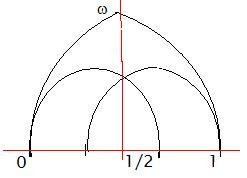The three pentagons on the upper half plane (ie. the front of the pair of pants) are marked by boundary pieces from the fundamental domain tesselation of the modular group. The compact pentagon has three edges on the real axis as shown, where the point $\omega$ is the vertex where the three pentagons meet. The real points -1 and 2 define the ends of the larger circles on the ribbon graph. Thus there exist modular transformations mapping points from one pentagon into the others. We would like to consider a triality for the pentagons, such as that given by $(ST)^{3} = 1$, which fixes $\omega$.

Recall also that the three strand braid group $B_3$, which covers the modular group, is the fundamental group for the complement of a trefoil knot in $\mathbb{R}^{3}$. Embedding the Riemann sphere in $\mathbb{R}^{3}$, we can draw the trefoil on the sphere and choose to place the three knot crossings near the points $0$, $1$ and $\infty$, which are the squares of the associahedron. The cell complex dual to this associahedron in $\mathbb{R}^{3}$ turned up in the description of the moduli for the 6-valent ribbon vertex of Mulase et al.

Aside: The critical line of the Riemann zeta function, which is self dual under the functional relation, is the line that divides the hexagon into two pentagons on the front of the pair of pants.

## Sunday, February 03, 2008

### M Theory Lesson 153

On the pair of pants, the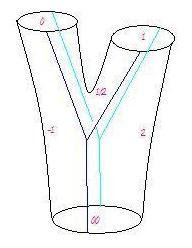hexagon on one side is divided into three pentagons. This triple is associated to the three level quantum number, called mass. Recall that these become three heptagons on a tiling of the Klein quartic. There are 24 such tiles.

Observe how the so-called higher dimensions
of string theory are simply spaces spanned by quantum numbers: two for spin, three for mass, six for em charge and so on.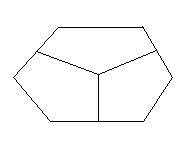In this light we can reevaluate ridiculous expressions of the form, "gravitons leak into extra dimensions". If a graviton is a particle, either it has certain quantum numbers or it doesn't. It cannot decide to take a leak and find itself more quantum numbers.

The 24 tiles are the 24 dimensions of the Leech lattice. The 8 hexagons are the 8 dimensions of the E8 lattice. Each of these exhibits a triality. I'm beginning to find this quite a lot of fun.

## Saturday, February 02, 2008

### M Theory Lesson 152

In a 2002 paper, in referring to another paper which never appeared, Batanin mentions a topological 2-operad containing the sequence of permutohedra, which are labelled by extended 1-ordinal trees as shown. The sequence begins with the interval, the hexagon and the hexagonal and square faced 24 vertex polytope.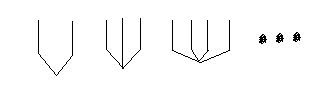Notice that the 2-ordinal labelling here is different from the more modern one, which Batanin used to solve the general compactification problem. For example, the hexagon should be replaced by the Breen polytope, a double 12 sided object, which may be resolved into a 12 sided cylinder in the B operad.

But sticking with the old example, the last polytope (labelled by the 4 leaved tree) maps to the 3d Stasheff associahedron (labelled by a single level 4 leaved tree) under a Loday type map, which forgets the levels on the trees that are used to label permutations. So the Loday-Ronco triples are based on 1-ordinal sequences, whereas we would like to view the permutohedra as part of a 2-operad, and similarly the cubes as part of a 3-operad. The old example actually considers a 2-operad in Cat, and another operad in Span(Cat) (spans in the category of categories), the algebras of which give the sought after Gray categories. If anyone has further references to such examples, I would really appreciate finding them!

Now a 3-ordinal tree with only three leaves, which looks like a central extension of the 2-ordinal tree which usually labels the hexagon, happens to label a hexagon of the form shown,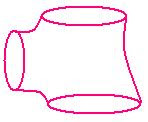which came up recently in lessons when we tried to tile Riemann surfaces with associahedra. So maybe this silly hexagon on a pair of pants really is trying to tell us something. We know we want it to come from a 3-operad eventually, because mass generation is characterised by Gray type structures.

## Friday, February 01, 2008

### M Theory Lesson 151

L. B. Crowell has shown up at PF with some remarks about sphere packing, codes and quantum gravity. He seems quite interested in the Leech lattice and associated theta functions, as well as the familiar j-invariant. The triple of $E8$ lattices is most evident in the generating function for the Leech theta series, namely

$f(q) = (\Theta (q))^{3} - 720 q^2 \prod_{1}^{\infty} (1 - q^{2n})^{24}$

where $\Theta (q)$ is the series for the $E8$ lattice. A triple $E8$ is suggestive from a traditional stringy point of view, extending the heterotic pair of $E8$ to a ternary logic. However, as Gannon explains, there is a more interesting triality involving affine $E8$, $F4$ and $G2$. One can label the $E8$ diagram by conjugacy classes of the Monster group! The $F4$ comes from a two folding of affine $E7$ and the $G2$ from a triple folding of affine $E6$. The $G2$ case corresponds to conjugacy classes for a Fischer group, which itself has a triple cover in one of the conjugacy classes of the Monster.

$\Theta$ is really the Eisenstein series $E_{4}$. The series $E_{2}(\sqrt{z})$, $E_{4}(\sqrt{z})$ and $E_{6}(\sqrt{z})$ satisfy the triality, for $D = z \frac{d}{dz}$,

$D E_{2} = \frac{1}{12} (E_{2}^{2} - E_{4})$
$D E_{4} = \frac{1}{3} (E_{2}E_{4} - E_{6})$
$D E_{6} = \frac{1}{2} (E_{2}E_{6} - E_{4}^{2})$

This triality uses the zeta values $\zeta (2)$, $\zeta (4)$ and $\zeta (6)$. The next Eisenstein series, $E_{8}$, corresponds to the theta series for $E8 \oplus E8$. The investigation of trialities for mass generation always seems to come back to this very fundamental mathematics.